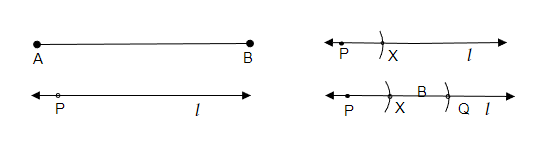# Given some line segment $\overline{\mathrm{AB}}$, whose length you do not know, construct $\overline{\mathrm{PQ}}$ such that the length of $\overline{\mathrm{PQ}}$ is twice that of $\overline{\mathrm{AB}}$.

Given:

A line segment $\overline{AB}$.

To do:

We have to construct $\overline{PQ}$ such that the length of $\overline{PQ}$ is twice that of $\overline{AB}$.

Solution:Steps of construction:

(i) To draw the line segment $\overline{PQ}$.

(ii) Let us place the pointer of the compasses on point A of the given line segment AB and adjust the compasses up to the length of $\overline{AB}$.

(iii) Let us draw a line 'l' and point a point P on the line.

(iv) Now, by placing the pointer of the compasses on point p let us cut the line 'l' and point it as X.

(v) Again, by pointing the pointer of the compasses on the point X draw another arc on the line 'l' and point it as Q.

(vi) Therefore, the required line segment $\overline{PQ}$ is formed.

Updated on: 10-Oct-2022

37 Views